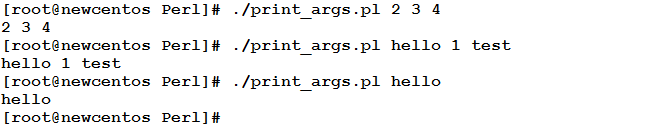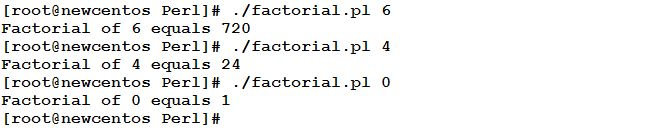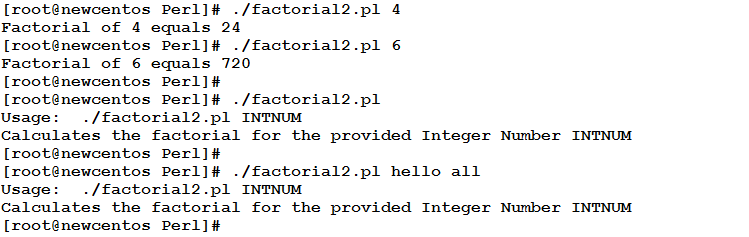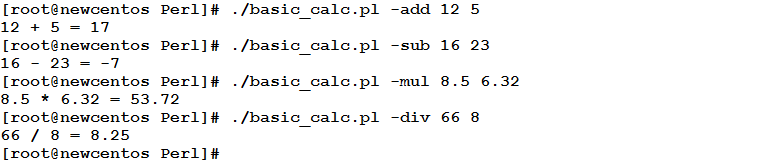# Learn to Use Command Line Arguments in Perl Programming

0
10146Command-Line arguments are inputs to the program, provided by the user on program execution. Using Command-Line Arguments is the subject of today’s article. So, be with us, and you won’t regret it!

The @ARGV Array
Perl has a special array @ARGV that contains the list of command-line arguments given to the program at execution.

Example
The following script prints the command-line arguments provided to it.

```#!/usr/bin/perl
#This program prints the provided command-line arguments.
#Author: Eduonix
#Date: Nov 2015
print ("@ARGV\n");
```

When executed, the program will act like this:So, the first element of the @ARGV array contains the first argument (if provided), the second element contains the second argument (if provided), and etc.
Consequently, the number of provided arguments to a script is equal to the length of @ARGV array.

Example
Let’s re-write the factorial script to take its input from the command-line instead of prompting the user to enter it.

```#!/usr/bin/perl
#This script accepts an integer as a command line argument
#and calculates its factorial.
##Author: Eduonix
##Date: Nov 2015
\$result = 1;
foreach \$i (1..\$ARGV)
{
\$result *= \$i;
}
print("Factorial of \$ARGV equals \$result\n");
```

Let’s see it in action:Special Variables
Besides to the default variable \$_ (that we introduced when talking about the foreach loop in Article (7) – Loops (1)) and the @ARGV array that stores the command-line arguments, Perl maintains a set of special variables that could be very useful in some situations. In this section, we are going to introduce some of these variables and their uses.

\$0 Variable
The \$0 variable contains the program name.

Example
Re-write the factorial program to verify that the user has provided exactly one argument. If not, the program should print a message like the following:

```Usage:	PROGRAM_NAME INTNUM
Calculates the factorial for the provided INTNUM
```

Consider the following code:

```#!/usr/bin/perl
#This script accepts an integer as a command line argument
#and calculates its factorial.
##Author: Eduonix
##Date: Nov 2015
if (scalar (@ARGV) == 1)
{
\$result = 1;
foreach \$i (1..\$ARGV)
{
\$result *= \$i;
}
print("Factorial of \$ARGV equals \$result\n");
}
else
{
print("Usage:\t\$0 INTNUM
Calculates the factorial for the provided Integer Number INTNUM\n");
}
```

When executed, this script should give the output:Despite its simplicity, I would like to explain two points:

• The expression scalar(@ARGV) returns the number of elements in the @ARGV array (which we know that it represents the number of typed arguments). Consequently, the if statement if (scalar (@ARGV) == 1) checks whether the user has provided only one argument.
• To print the script name in the usage message, we used the \$0 variable.The Default Array @_
The array @_ contains the list of arguments passed to a subroutine. This will be discussed in detail in the next article “Subroutines”.

Using Command-Line Options
Similar to the system commands that accept command-line options (switches) that control the command behavior, Perl scripts could also accept command-line options.

For the Perl interpreter to be able to parse the command-line switches, it needs to be invoked with the –s option. When the interpreter encounters a switch like for example –new, it will expect to see (within the script) a variable called \$new. When seen, the variable \$new will be set to true. If the command-line switch uses a form like for example –num=23, the Perl interpreter will set the variable \$num to 23.

Example
Write a basic calculator program that expects three command-line arguments as follows:

• An option that determines the required operation such as –add, -sub, -mul, or –div
• Two numbers that represent the required operands for the arithmetic operation.

Consider the following code:

```#!/usr/bin/perl -s
#Author: Eduonix
#Date: Oct 2015
{
print ("\$ARGV + \$ARGV = ", \$ARGV + \$ARGV,"\n");
}
elsif (\$sub)
{
print ("\$ARGV - \$ARGV = ", \$ARGV - \$ARGV,"\n");
}
elsif (\$mul)
{
print ("\$ARGV * \$ARGV = ", \$ARGV * \$ARGV,"\n");
}
elsif (\$div)
{
print ("\$ARGV / \$ARGV = ", \$ARGV / \$ARGV,"\n");
}
```

When executed, the script will act as follows:As you can see, the shebang uses the –s option to enable interpreting command-line options. When the script is executed with –add option, the variable \$add will be true, and hence the if(\$add) condition will be met, and consequently: its body will be executed.

Summary